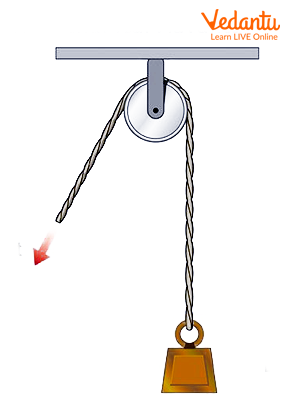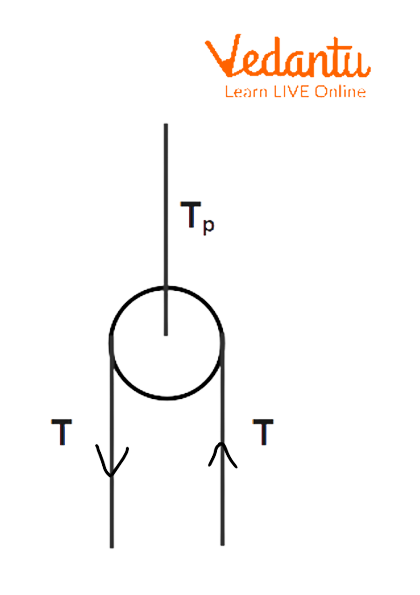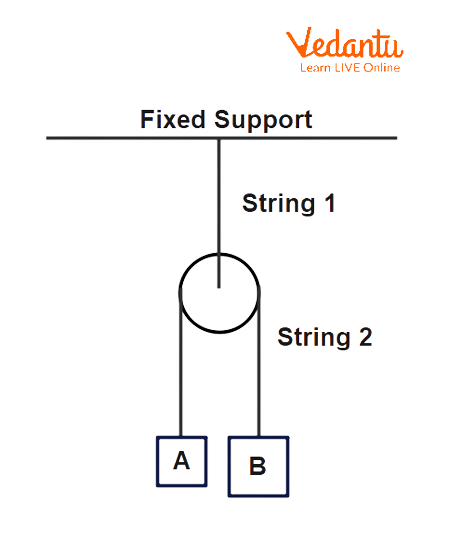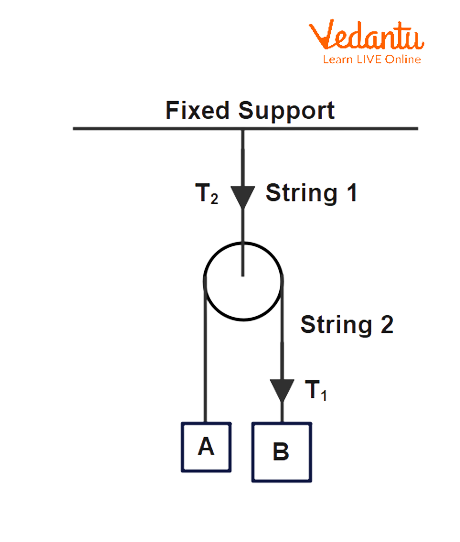Courses
Courses for Kids
Free study material
Free LIVE classes
More

# Pulley and Constraint Relations - JEE Important TopicLIVE
Join Vedantu’s FREE Mastercalss

## An Introduction to Pulley and Constraints

We all have seen pulleys in our day-to-day life and also sometimes used them in various places such as well for pulling water. It is a very powerful method which includes a wheel and a string and uses small force to pull large objects. But we all know that there are some forces such as gravity or friction which restrict or oppose the motion of any body. In classical mechanics, these restrictions are called constraints. The type of motion which has constraints is called constraint motion.

In this article, we are going to understand pulley, constraints, relation between pulley and constraints, and problems related to pulley and constraints relation.

## What is a Pulley?

A pulley is defined as a machine made up of metal or wood which uses a wheel and a rope for lifting heavy objects by applying small force. A pulley works when a force is applied on one side of the rope while the other side is connected to a heavy weight which needs to be pulled. On applying force, the rope moves downward through the wheel and the weight on the other side gets pulled up. This phenomenon is commonly used in pulling water from the wells.Pulley System

## What are Constraints?

The restrictions or opposition to the motion of any object caused by different factors which reduce its free movement are called constraints. The type of motion which has constraints is called constraint motion.

According to the constraint relation, the sum of products of all tensions in the strings and velocities of blocks connected to the strings is equal to 0. Also, it can be said that the total power by tension is 0. This relation can be represented as follows:

$\sum{T.\text{ }\overline{v}=0}$

If we differentiate the above equation with respect to time while keeping the velocity vector constant, we get another relation which is given as

$\sum{T.\text{ }\overline{a}=0}$

This is so because, when a velocity vector is kept constant, the sum of products of all tensions in the strings and the accelerations of blocks connected to the strings is equal to 0.

## Relation Between Pulley and Constraints

According to the pulley and constraints relation, when it is given that the pulley is massless, then the tensions present on both the sides of the pulley are equal. This relation can be represented as follows:

$2{{T}_{P}}=T+T=2T$Pulley with Tensions in Strings

## Problems Related to Pulley and Constraint Relation

Problem-1: It is given that the pulley in the below diagram is massless and the mass of block A and B is 7kg and 9kg, respectively. Calculate the acceleration of the two blocks and tensions in string 1 and string 2.Pulley with Masses A and B

Solution: The tensions in the strings are depicted below.Pulley with Masses A and B with tension T1 and T2

Now, let $a$ be the acceleration of the two blocks and ${{T}_{1}}$ and ${{T}_{2}}$ be the tensions in the strings 1 and 2, respectively.

The formula for tension is

$T = mg + ma$

So, the tension in string 1 due to block A is

${{T}_{1}} = 7\times 10+7\times a$

${{T}_{1}} = 70 + 7a$

And, the tension in string 1 due to block B is

${{T}_{1}} = 9\times 10 + 9\times a$

${{T}_{1}} = 90 + 9a$

Also,

${{T}_{1}} - 70 = 7a$ and $90- {{T}_{1}} = 9a$

On subtracting both the equations, we get

$20 = 16a$

$a = 1.25mlse{{c}^{2}}$

Now, substitute the value of a in any of the equations.

${{T}_{1}} = 7 + 7\times 1.25$

${{T}_{1}} = 96.25N$

As the given pulley is massless,

${{T}_{2}} = 2{{T}_{1}}$

Thus,

${{T}_{2}} = 192.5N$

## Summary

A pulley is a machine made up of metal or wood which uses a wheel and a rope for lifting heavy objects by applying small force. The restrictions on the motion of any object caused by different factors which reduce its free movement are called constraints. The pulley and constraints relation is given by the equation $2{{T}_{P}} = T + T = 2T$. In order to calculate the acceleration or tension in two blocks connected to a pulley, first use the formula of tension and then use the pulley constraint relation. On further solving, the acceleration and tension of the two blocks can be calculated.

Last updated date: 16th Sep 2023
Total views: 115.5k
Views today: 4.15k

## FAQs on Pulley and Constraint Relations - JEE Important Topic

1. Define pulley.

A pulley is defined as a machine made up of metal or wood which uses a wheel and a rope for lifting heavy objects by applying small force. A pulley works when a force is applied on one side of the rope while the other side is connected to a heavy weight which needs to be pulled. On applying force, the rope moves downward through the wheel and the weight on the other side gets pulled up. This phenomenon is commonly used in pulling water from the wells.

2. Give any four everyday examples of the pulley.

Some everyday examples of pulley include the following:

• Lift uses a pulley to pull the passengers.

• Theatre curtains use pulley for pulling and dropping down the curtains.

• Gym equipment also contain pulley for exercises.

• Construction

3. What are constraints? Also, explain constraints relation.

The restrictions or opposition on the motion of any object caused by different factors which reduces its free movement are called constraints. The type of motion which has constraints is called constraint motion.